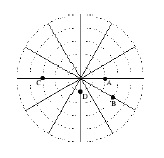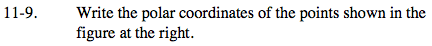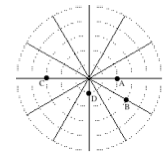Home > PC > Chapter 11 > Lesson 11.1.1 > Problem11-9

11-9.
1. Write the polar coordinates of the points shown in the figure below. Homework Help ✎

2.Identify the radius and angle of rotation for each point.

$B=\left(3,\frac{11\pi}{6} \right)=\left( 3,-\frac{\pi}{6} \right)$